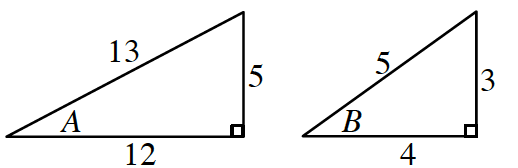### Home > PC3 > Chapter 10 > Lesson 10.2.1 > Problem10-115

10-115.

If $\sin(A) = \frac { 5 } { 13 }$ and $\sec(B) =\frac { 5 } { 4 }$, where $0 < A, B < \frac { \pi } { 2 }$, determine the exact value of:

1. $\cos(A)$

1. $\sin(B)$

1. $\cos(A + B)$$\cos(A + B) = \cos^2(A) − \cos^2(B)$